Скачать презентацию Physics 1501 Lecture 3 Today s Agenda l Announcements

865db3284cba6a1fac0ee2b8a9c77f0d.ppt

• Количество слайдов: 30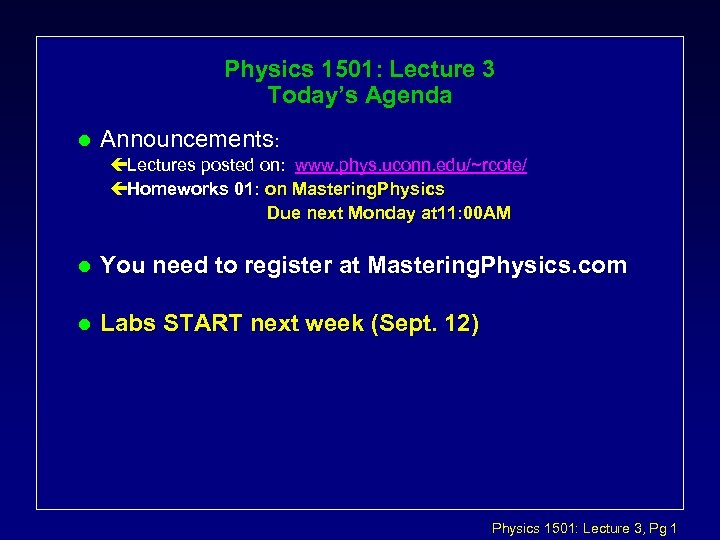Physics 1501: Lecture 3 Today’s Agenda l Announcements: çLectures posted on: www. phys. uconn. edu/~rcote/ çHomeworks 01: on Mastering. Physics Due next Monday at 11: 00 AM l You need to register at Mastering. Physics. com l Labs START next week (Sept. 12) Physics 1501: Lecture 3, Pg 1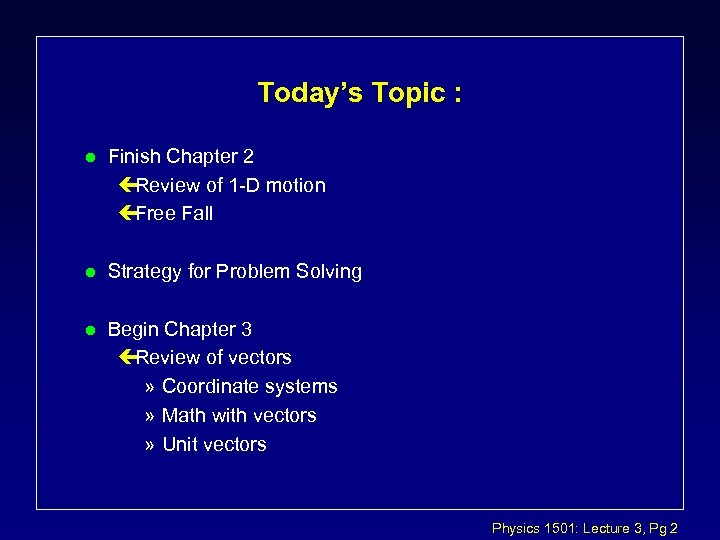Today’s Topic : l Finish Chapter 2 çReview of 1 -D motion çFree Fall l Strategy for Problem Solving l Begin Chapter 3 çReview of vectors » Coordinate systems » Math with vectors » Unit vectors Physics 1501: Lecture 3, Pg 2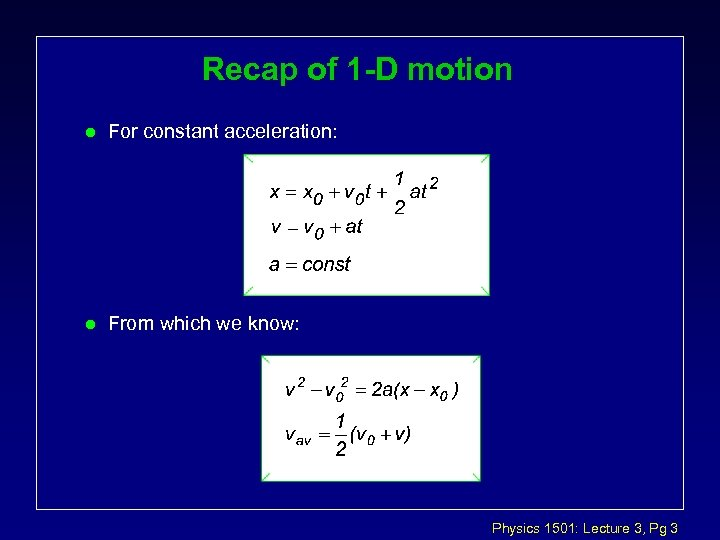Recap of 1 -D motion l For constant acceleration: l From which we know: Physics 1501: Lecture 3, Pg 3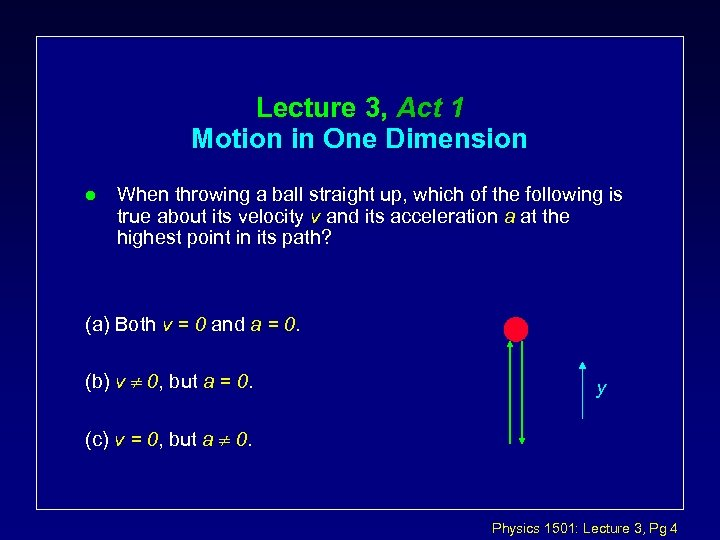Lecture 3, Act 1 Motion in One Dimension l When throwing a ball straight up, which of the following is true about its velocity v and its acceleration a at the highest point in its path? (a) Both v = 0 and a = 0. (b) v 0, but a = 0. y (c) v = 0, but a 0. Physics 1501: Lecture 3, Pg 4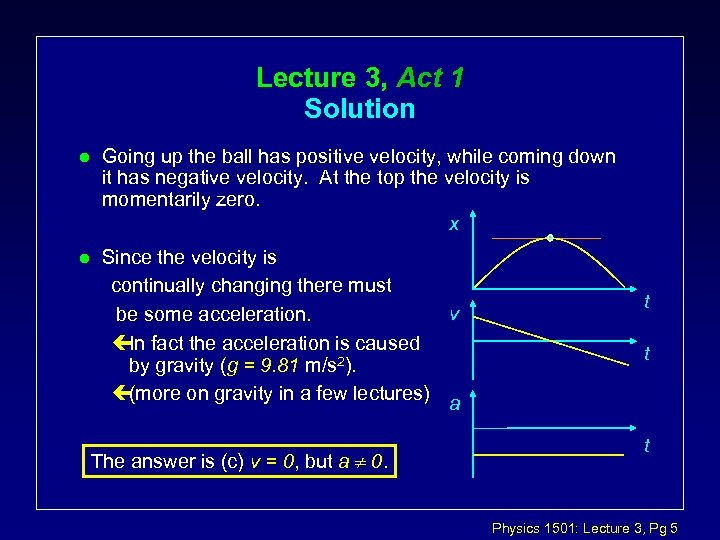Lecture 3, Act 1 Solution l Going up the ball has positive velocity, while coming down it has negative velocity. At the top the velocity is momentarily zero. x l Since the velocity is continually changing there must v be some acceleration. çIn fact the acceleration is caused by gravity (g = 9. 81 m/s 2). ç(more on gravity in a few lectures) a The answer is (c) v = 0, but a 0. t t t Physics 1501: Lecture 3, Pg 5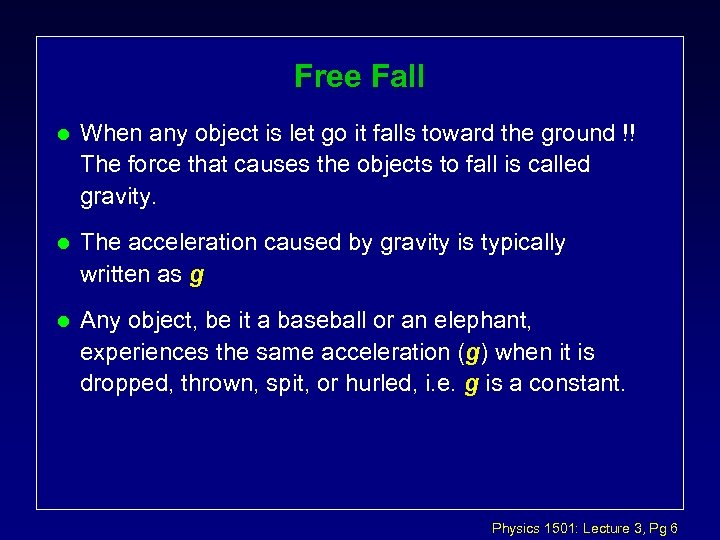Free Fall l When any object is let go it falls toward the ground !! The force that causes the objects to fall is called gravity. l The acceleration caused by gravity is typically written as g l Any object, be it a baseball or an elephant, experiences the same acceleration (g) when it is dropped, thrown, spit, or hurled, i. e. g is a constant. Physics 1501: Lecture 3, Pg 6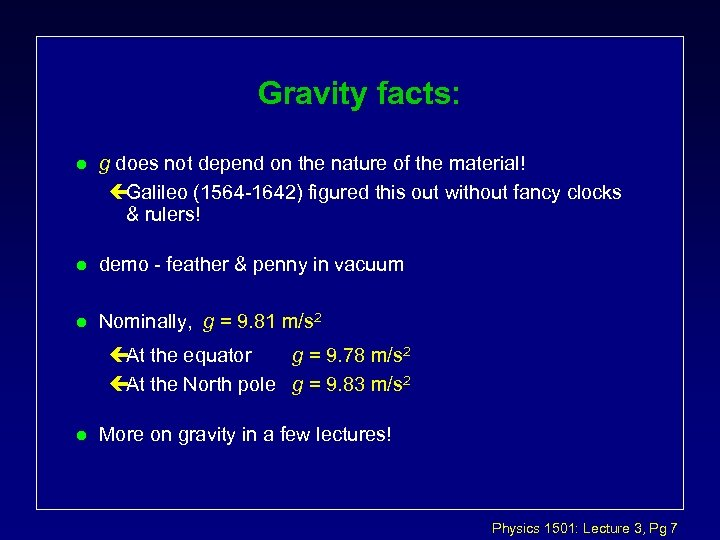Gravity facts: l g does not depend on the nature of the material! çGalileo (1564 -1642) figured this out without fancy clocks & rulers! l demo - feather & penny in vacuum l Nominally, g = 9. 81 m/s 2 çAt the equator g = 9. 78 m/s 2 çAt the North pole g = 9. 83 m/s 2 l More on gravity in a few lectures! Physics 1501: Lecture 3, Pg 7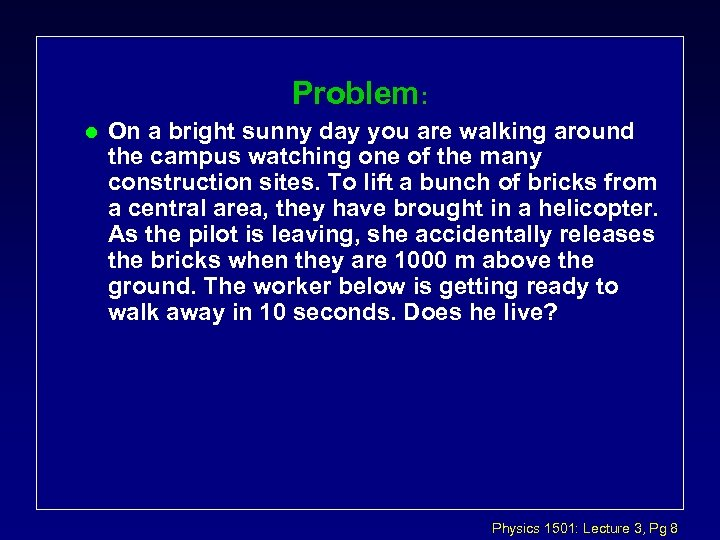Problem: l On a bright sunny day you are walking around the campus watching one of the many construction sites. To lift a bunch of bricks from a central area, they have brought in a helicopter. As the pilot is leaving, she accidentally releases the bricks when they are 1000 m above the ground. The worker below is getting ready to walk away in 10 seconds. Does he live? Physics 1501: Lecture 3, Pg 8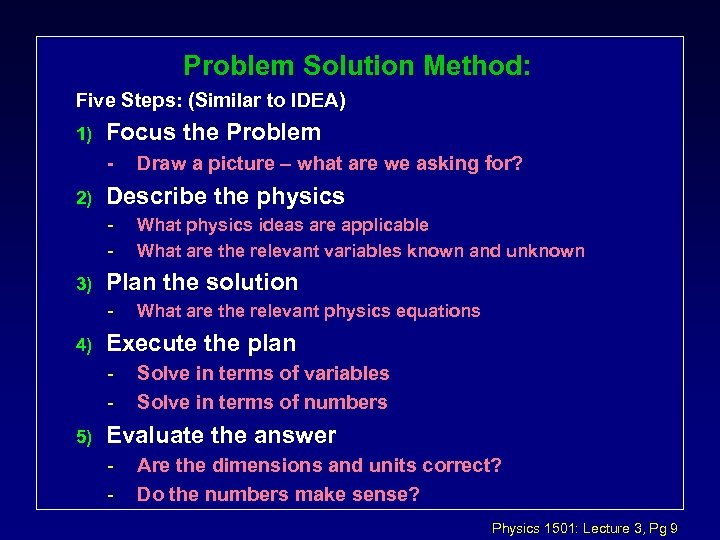Problem Solution Method: Five Steps: (Similar to IDEA) 1) Focus the Problem - 2) Describe the physics - 3) What are the relevant physics equations Execute the plan - 5) What physics ideas are applicable What are the relevant variables known and unknown Plan the solution - 4) Draw a picture – what are we asking for? Solve in terms of variables Solve in terms of numbers Evaluate the answer - Are the dimensions and units correct? Do the numbers make sense? Physics 1501: Lecture 3, Pg 9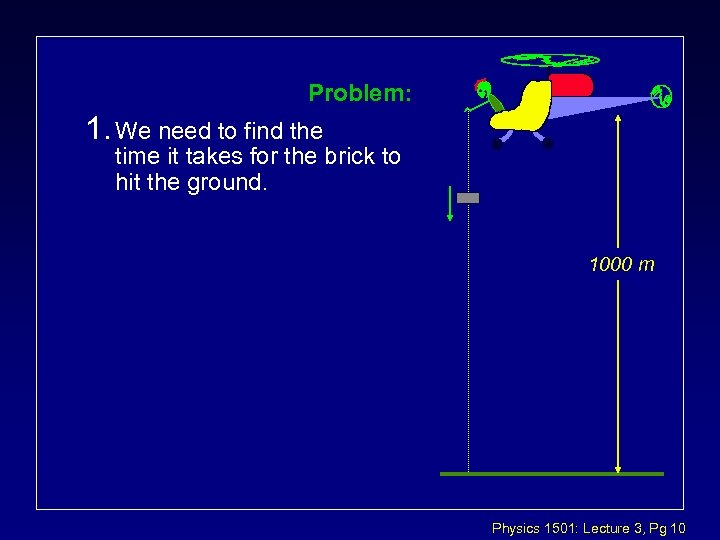Problem: 1. We need to find the time it takes for the brick to hit the ground. 1000 m Physics 1501: Lecture 3, Pg 10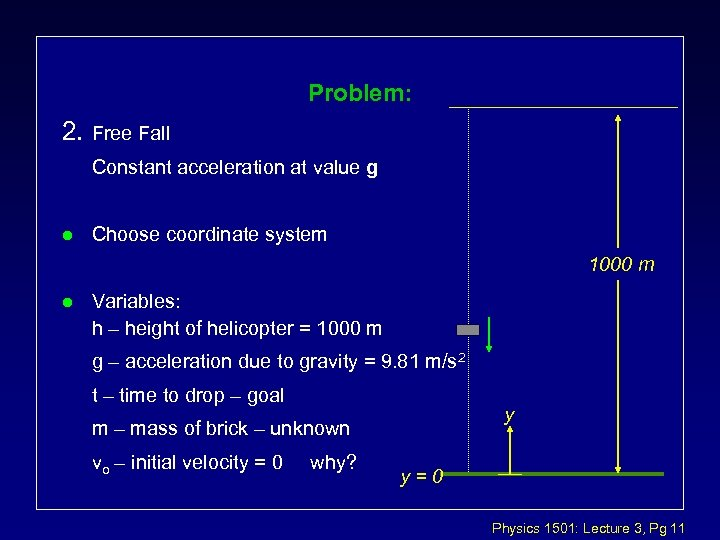Problem: 2. Free Fall Constant acceleration at value g l Choose coordinate system 1000 m l Variables: h – height of helicopter = 1000 m g – acceleration due to gravity = 9. 81 m/s 2 t – time to drop – goal y m – mass of brick – unknown vo – initial velocity = 0 why? y=0 Physics 1501: Lecture 3, Pg 11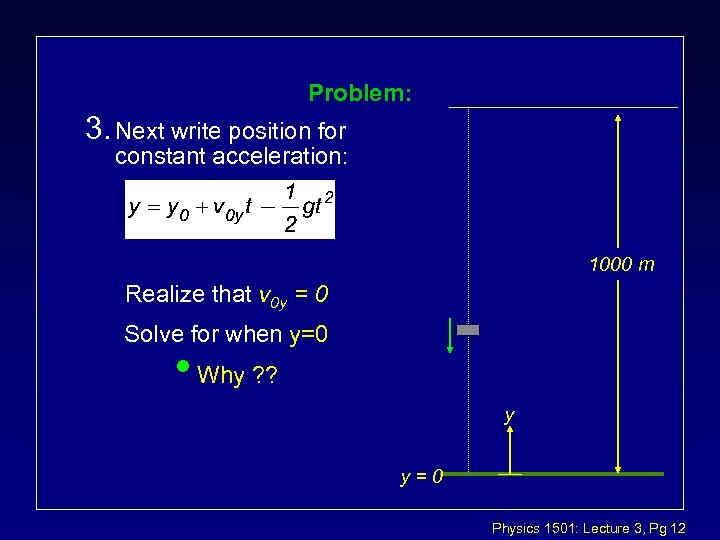Problem: 3. Next write position for constant acceleration: 1000 m Realize that v 0 y = 0 Solve for when y=0 • Why ? ? y y=0 Physics 1501: Lecture 3, Pg 12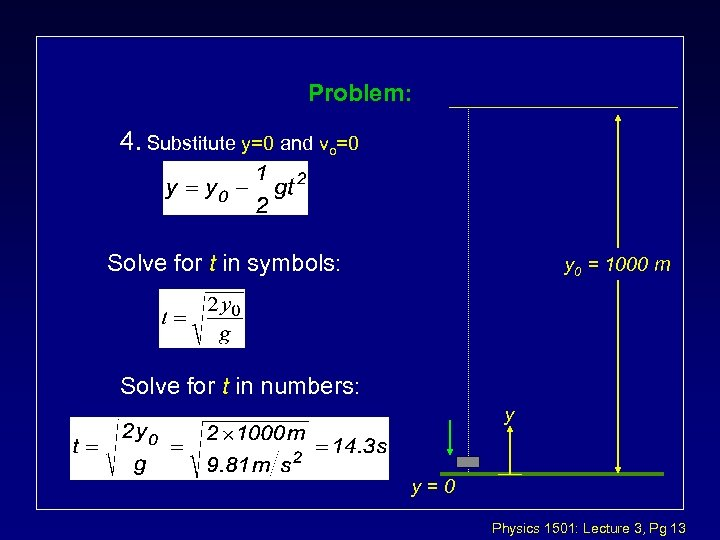Problem: 4. Substitute y=0 and vo=0 Solve for t in symbols: y 0 = 1000 m Solve for t in numbers: y y=0 Physics 1501: Lecture 3, Pg 13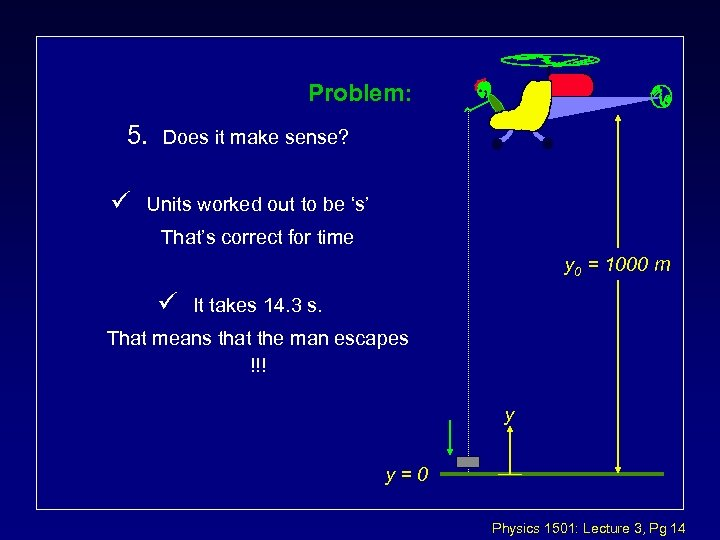Problem: 5. ü Does it make sense? Units worked out to be ‘s’ That’s correct for time y 0 = 1000 m ü It takes 14. 3 s. That means that the man escapes !!! y y=0 Physics 1501: Lecture 3, Pg 14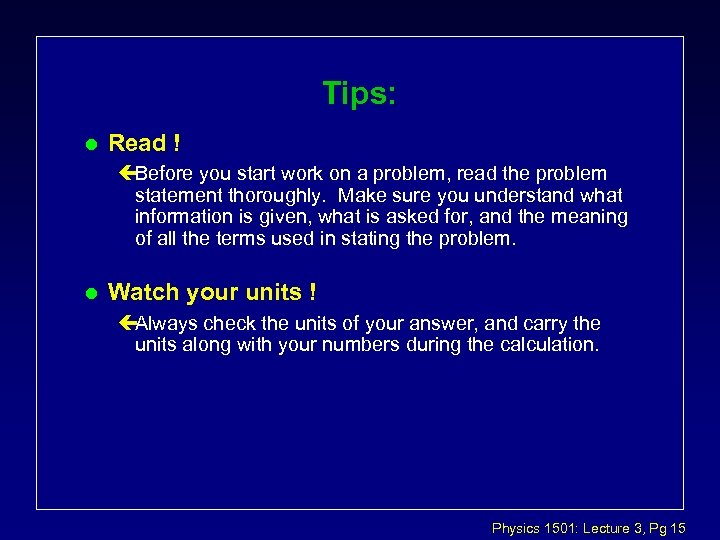Tips: l Read ! çBefore you start work on a problem, read the problem statement thoroughly. Make sure you understand what information is given, what is asked for, and the meaning of all the terms used in stating the problem. l Watch your units ! çAlways check the units of your answer, and carry the units along with your numbers during the calculation. Physics 1501: Lecture 3, Pg 15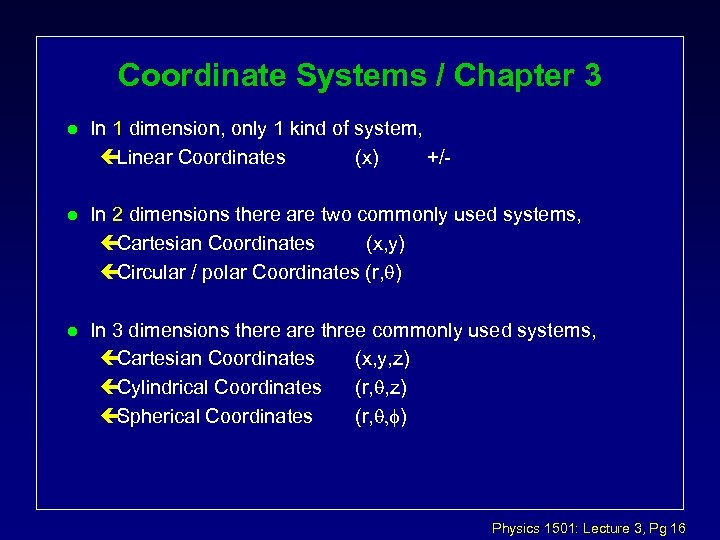Coordinate Systems / Chapter 3 l In 1 dimension, only 1 kind of system, çLinear Coordinates (x) +/- l In 2 dimensions there are two commonly used systems, çCartesian Coordinates (x, y) çCircular / polar Coordinates (r, ) l In 3 dimensions there are three commonly used systems, çCartesian Coordinates (x, y, z) çCylindrical Coordinates (r, , z) çSpherical Coordinates (r, , f) Physics 1501: Lecture 3, Pg 16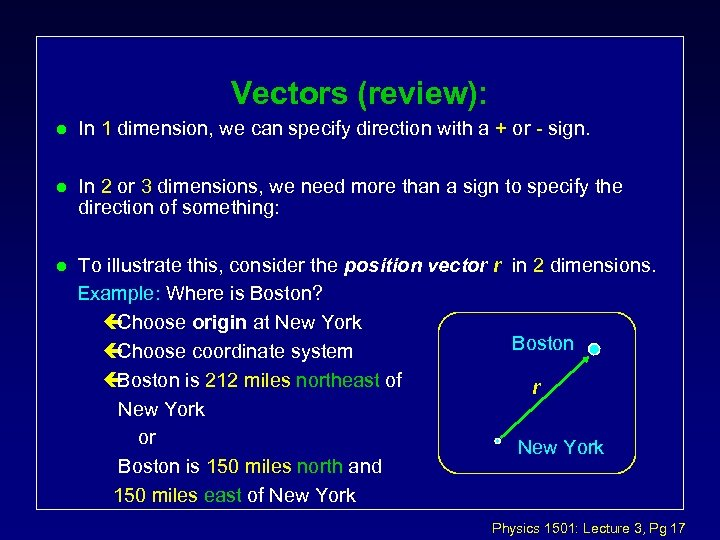Vectors (review): l In 1 dimension, we can specify direction with a + or - sign. l In 2 or 3 dimensions, we need more than a sign to specify the direction of something: l To illustrate this, consider the position vector r in 2 dimensions. Example: Where is Boston? Example ç Choose origin at New York Boston ç Choose coordinate system ç Boston is 212 miles northeast of r New York or New York Boston is 150 miles north and 150 miles east of New York Physics 1501: Lecture 3, Pg 17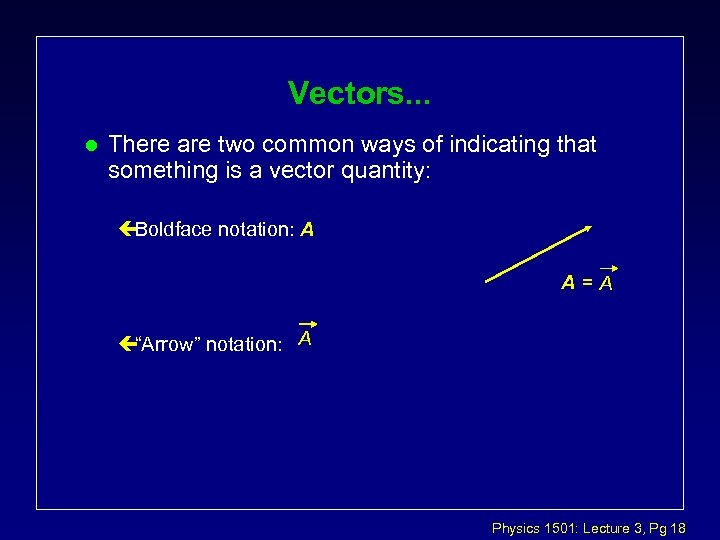Vectors. . . l There are two common ways of indicating that something is a vector quantity: çBoldface notation: A A=A ç“Arrow” notation: A Physics 1501: Lecture 3, Pg 18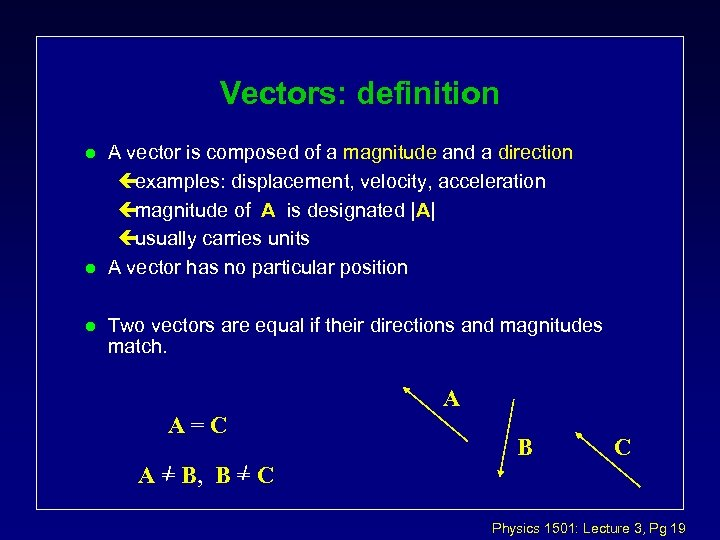Vectors: definition l l l A vector is composed of a magnitude and a direction çexamples: displacement, velocity, acceleration çmagnitude of A is designated |A| çusually carries units A vector has no particular position Two vectors are equal if their directions and magnitudes match. A A=C B C A = B, B = C Physics 1501: Lecture 3, Pg 19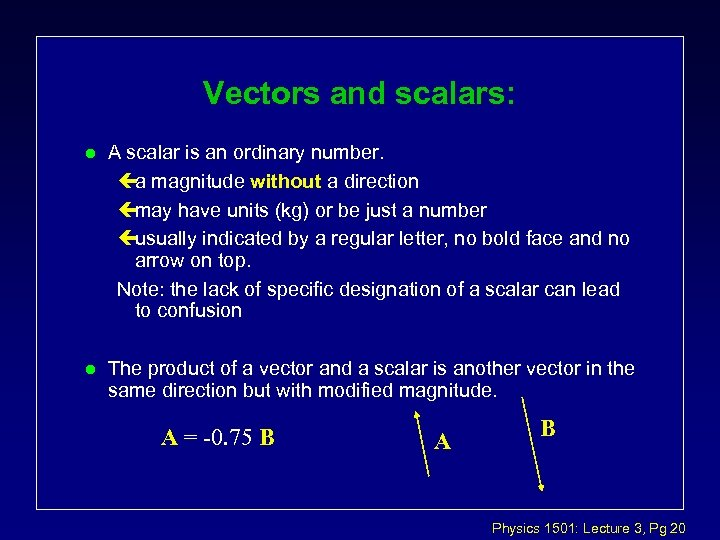Vectors and scalars: l A scalar is an ordinary number. ça magnitude without a direction çmay have units (kg) or be just a number çusually indicated by a regular letter, no bold face and no arrow on top. Note: the lack of specific designation of a scalar can lead to confusion l The product of a vector and a scalar is another vector in the same direction but with modified magnitude. A = -0. 75 B A B Physics 1501: Lecture 3, Pg 20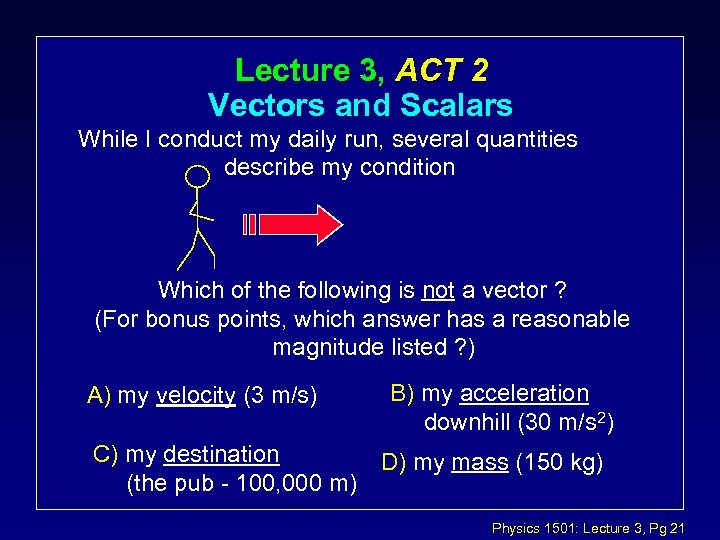Lecture 3, ACT 2 Vectors and Scalars While I conduct my daily run, several quantities describe my condition Which of the following is not a vector ? (For bonus points, which answer has a reasonable magnitude listed ? ) A) my velocity (3 m/s) C) my destination (the pub - 100, 000 m) B) my acceleration downhill (30 m/s 2) D) my mass (150 kg) Physics 1501: Lecture 3, Pg 21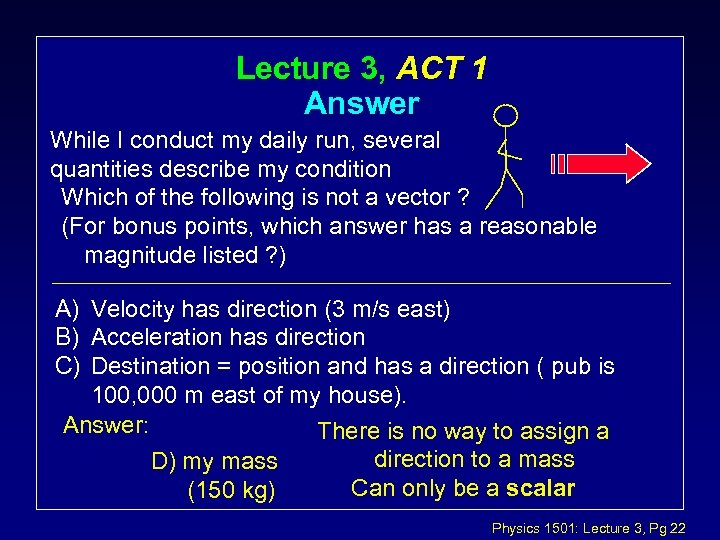Lecture 3, ACT 1 Answer While I conduct my daily run, several quantities describe my condition Which of the following is not a vector ? (For bonus points, which answer has a reasonable magnitude listed ? ) A) Velocity has direction (3 m/s east) B) Acceleration has direction C) Destination = position and has a direction ( pub is 100, 000 m east of my house). Answer: There is no way to assign a direction to a mass D) my mass Can only be a scalar (150 kg) Physics 1501: Lecture 3, Pg 22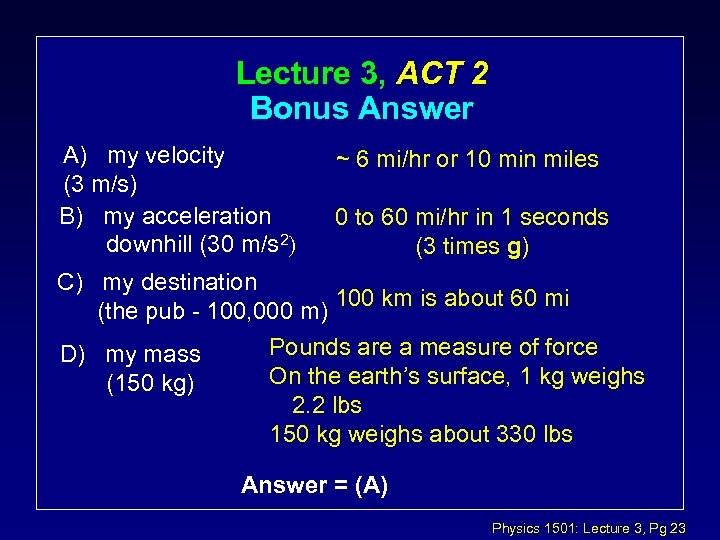Lecture 3, ACT 2 Bonus Answer A) my velocity (3 m/s) B) my acceleration downhill (30 m/s 2) ~ 6 mi/hr or 10 min miles 0 to 60 mi/hr in 1 seconds (3 times g) C) my destination 100 km is about 60 mi (the pub - 100, 000 m) D) my mass (150 kg) Pounds are a measure of force On the earth’s surface, 1 kg weighs 2. 2 lbs 150 kg weighs about 330 lbs Answer = (A) Physics 1501: Lecture 3, Pg 23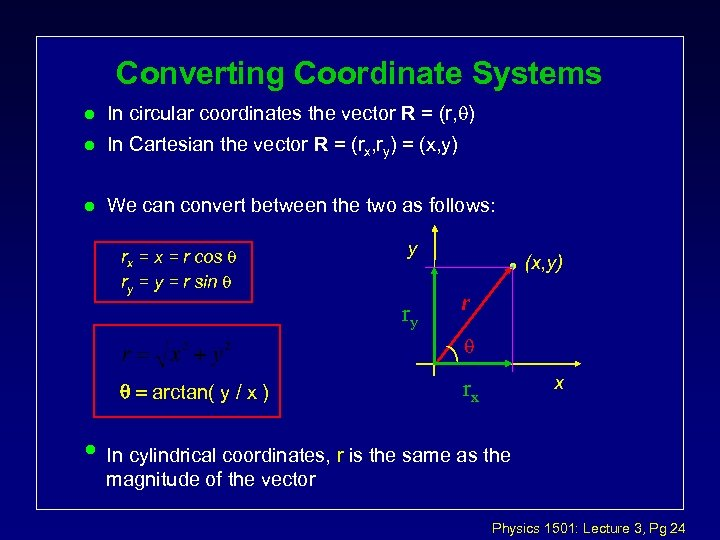Converting Coordinate Systems l In circular coordinates the vector R = (r, ) l In Cartesian the vector R = (rx, ry) = (x, y) l We can convert between the two as follows: rx = r cos ry = r sin y ry (x, y) r arctan( y / x ) • x rx In cylindrical coordinates, r is the same as the magnitude of the vector Physics 1501: Lecture 3, Pg 24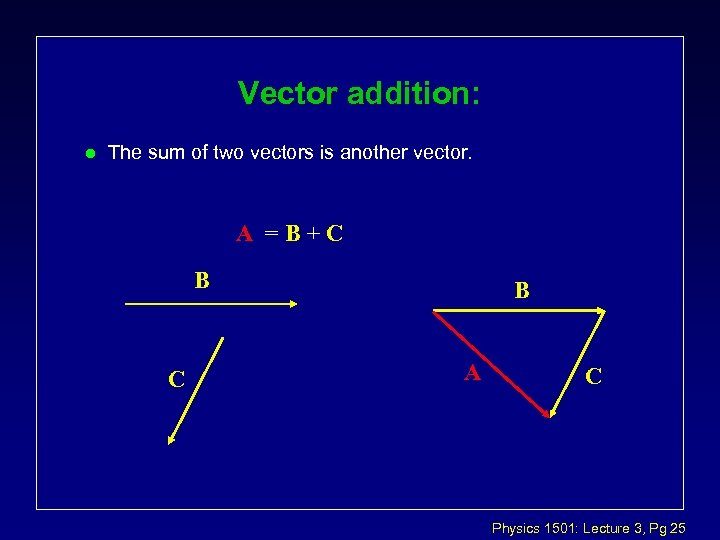Vector addition: l The sum of two vectors is another vector. A =B+C B A C Physics 1501: Lecture 3, Pg 25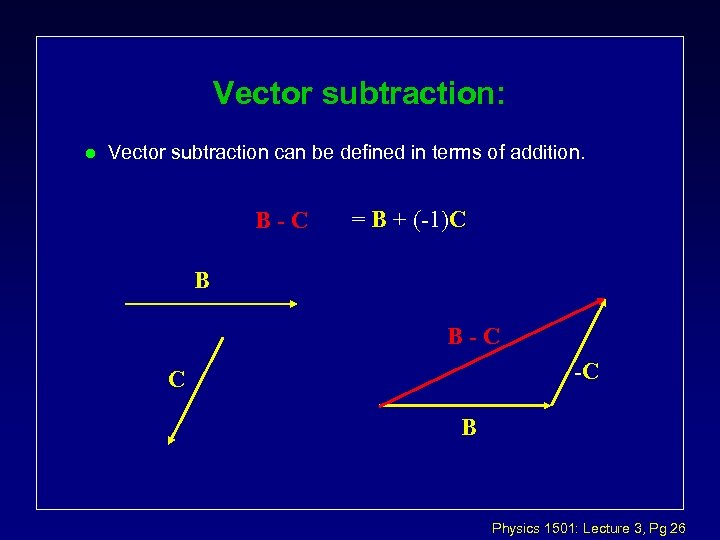Vector subtraction: l Vector subtraction can be defined in terms of addition. B-C = B + (-1)C B B-C -C C B Physics 1501: Lecture 3, Pg 26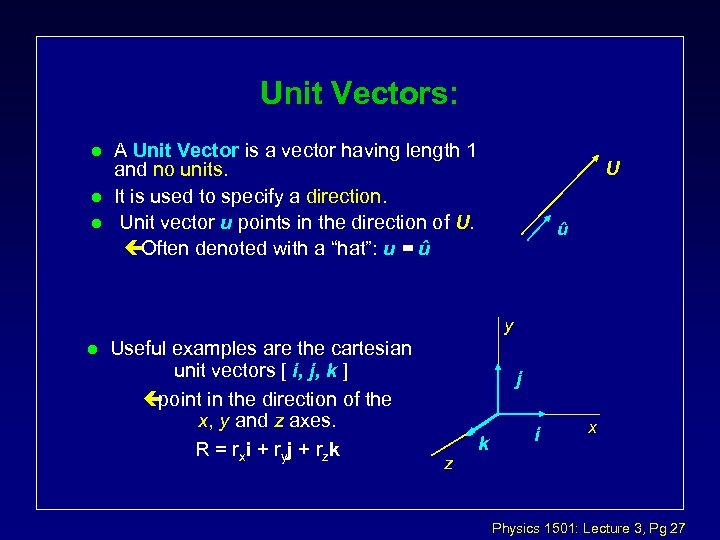Unit Vectors: l l l A Unit Vector is a vector having length 1 and no units. It is used to specify a direction. Unit vector u points in the direction of U. çOften denoted with a “hat”: u = û U û y l Useful examples are the cartesian unit vectors [ i, j, k ] ç point in the direction of the x, y and z axes. R = r xi + r yj + r zk j z k i x Physics 1501: Lecture 3, Pg 27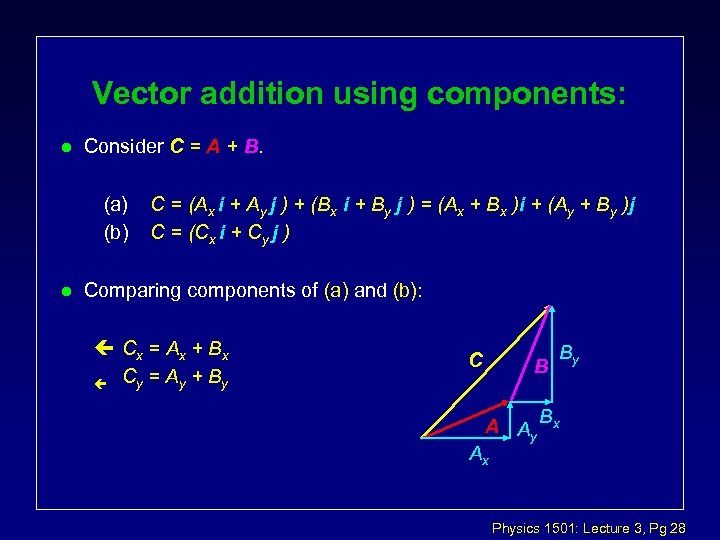Vector addition using components: l Consider C = A + B. (a) (b) l C = (Ax i + Ay j ) + (Bx i + By j ) = (Ax + Bx )i + (Ay + By )j C = (Cx i + Cy j ) Comparing components of (a) and (b): ç Cx = A x + B x ç Cy = A y + B y C B By A Ay Bx Ax Physics 1501: Lecture 3, Pg 28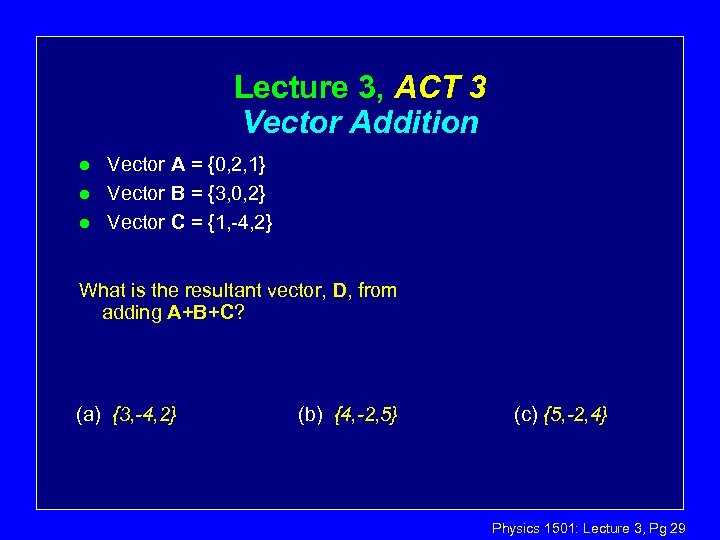Lecture 3, ACT 3 Vector Addition l l l Vector A = {0, 2, 1} Vector B = {3, 0, 2} Vector C = {1, -4, 2} What is the resultant vector, D, from adding A+B+C? (a) {3, -4, 2} (b) {4, -2, 5} (c) {5, -2, 4} Physics 1501: Lecture 3, Pg 29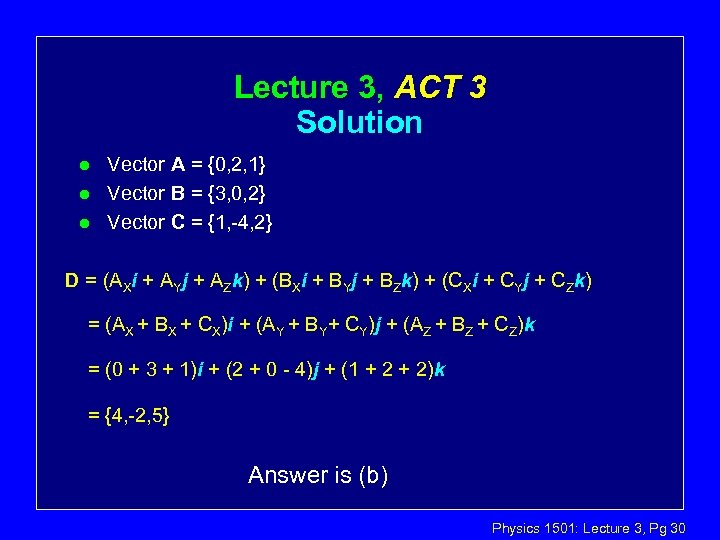Lecture 3, ACT 3 Solution l l l Vector A = {0, 2, 1} Vector B = {3, 0, 2} Vector C = {1, -4, 2} D = (AXi + AYj + AZk) + (BXi + BYj + BZk) + (CXi + CYj + CZk) = (AX + BX + CX)i + (AY + BY+ CY)j + (AZ + BZ + CZ)k = (0 + 3 + 1)i + (2 + 0 - 4)j + (1 + 2)k = {4, -2, 5} Answer is (b) Physics 1501: Lecture 3, Pg 30